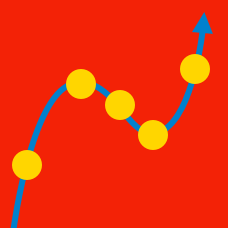Calculus

# Curve Sketching Warmup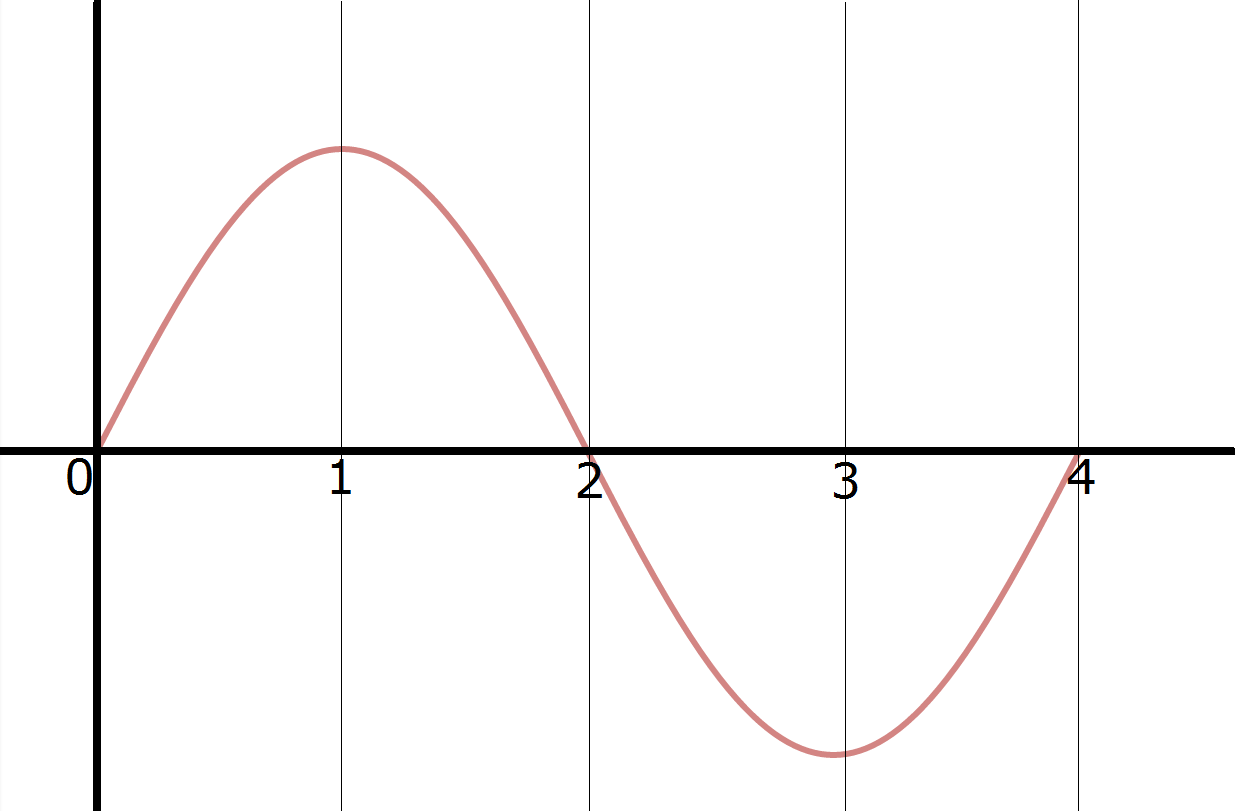The graph of $y = f(x)$ is shown. On which intervals is $f'(x) > 0?$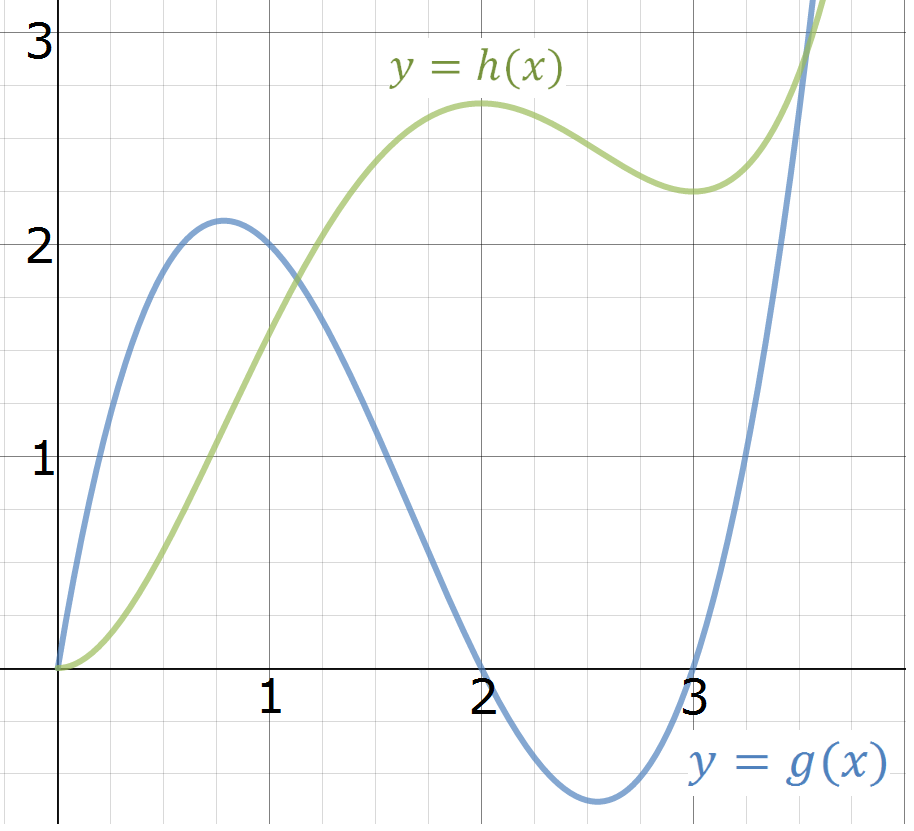The graphs of $y = g(x)$ and $y = h(x)$ are shown above. Which is the derivative of the other?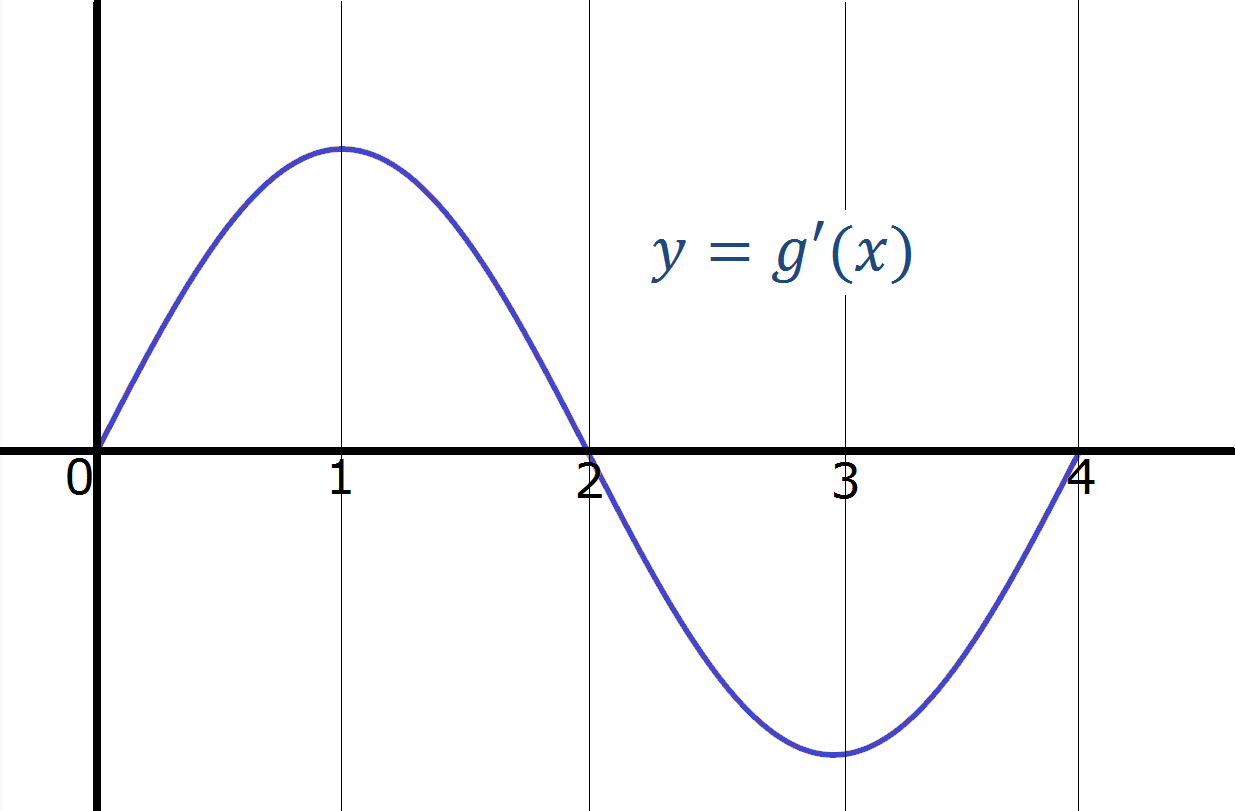The graph of $y = g'(x)$ (the derivative of $g(x)!)$ is shown. At what value of $x$ does $g(x)$ obtain a local maximum?

On what intervals is $f(x) = x^3 - 12x$ increasing?

$A = (-\infty, -2)$

$B = (-2, 2)$

$C = (2, \infty)$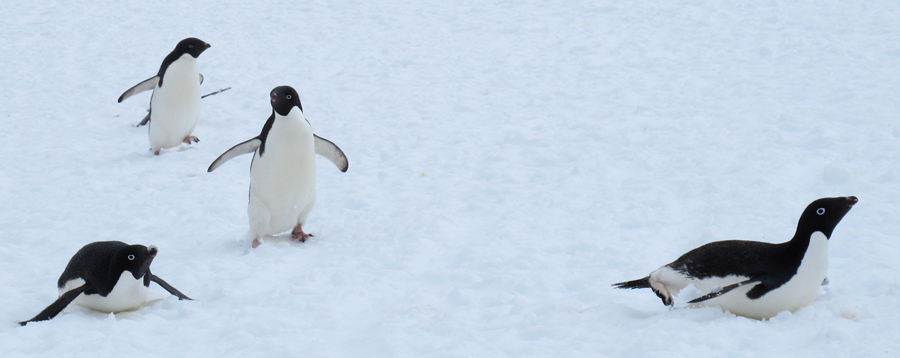A penguin is climbing up a long slippery slope, taking occasional breaks to slide back down for a bit. The penguin's vertical height (in meters) above a fixed point at time $t$ minutes after starting is represented by $f(t).$ Based on the information about $f'(t)$ in the table below, at which of these times did the penguin's height reach a local minimum?

 Interval Sign of $f'(t)$ $(0,1)$ + $(1,2)$ - $(2,3)$ - $(3,4)$ +
×